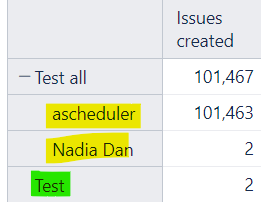# Alignment of calculated/non-calculated members

Hi,

I have 2 calculated members Test and Test all. Test all includes Test and other non-calculated members and there is a different alignment between Test (green) and the non-calculated members (yellow) when I expand Test all (see image below). Is it possible to change this so all are aligned?Test:
`Sum({[Assignee].[Nadia Dan]})`

Test all:
`Aggregate({ [Assignee].[ascheduler], [Assignee].[Nadia Dan], [Assignee].[Test] })`

Thanks,